## Operator PseudoRandomNumberGen

The operator PseudoRandomNumberGen generates a stream of N bit random numbers using linear feedback shift registers (LFSR). These LFSRs generate uniformly distributed binary random sequences. The length of the random sequence until it is repeated is defined by the number of registers of the feedback shift registers. A LFSR of 32 bit length will generate a sequence of 2^32 - 2 values. The implemented random number generator uses different LFSRs to generate the N bit random number at the operator output. This increases the sequence lengths and avoids correlations between the bits. The following figure illustrates the implementation of the pseudo random number generator.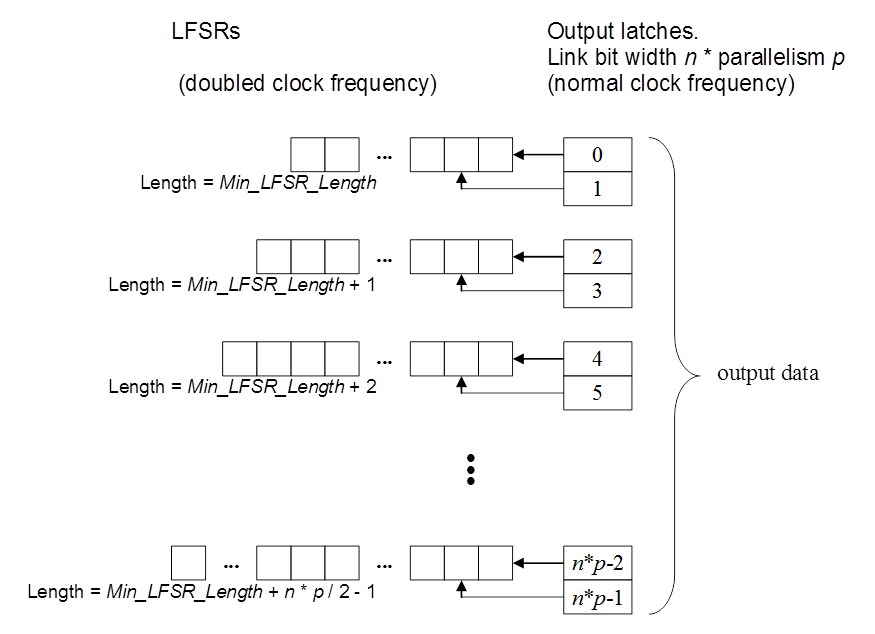To further improve the random number quality, the LFSRs are free running and not clock sourced by the pixel frequency. The timing of the results therefore is non-deterministic and hence, real random numbers are taken into the value generation which dramatically improves the quality.

The LFSRs are preinitialized with a seed on initialization of the applet. This seed is defined in VisualApplets using parameters Seed0, Seed1 and Seed2. On operator instantiation, a software random number generator is used for the default seed values.

### Usage

The operator supports different bit widths and parallelism. However, for high parallelism and bit width more resources are required. The output bit width can be adapted using the output link.

The operator input link is only used for synchronization. The data values on the input link are not used for data output generation.

Parameter Min_LFSR_Length is used to specify the length of the shortest LFSR. For each further LFSR another register stage is added. The maximum register length is 168.

To reduce the required resources of the operator, reduce parameter Min_LFSR_Length. If more than one operator is used with the same Min_LFSR_Length they will both generate the same sequence. If the seeds are different, the sequences are still the same, only the starting point of the sequence changed.

### Quality of the Generator Random Numbers

A pseudo random number generator can never generate real random values. Due to the efficient generator implementation and the adding of non-deterministic timings, the quality of the generated values is very high. In the following, the results of two tests applied on the generator are presented to prove the quality of the implementation.

1. Test on Equidistribution:

A sequence of 8 bit random values is generated and the mean value is determined. The mean value should be around 127.5. From theory of the LFSR we know that sequences are always equidistributed (except value 0 in all registers).

If looking at a histogram of the generated values (for example in microDisplay), the uniform distribution can be seen.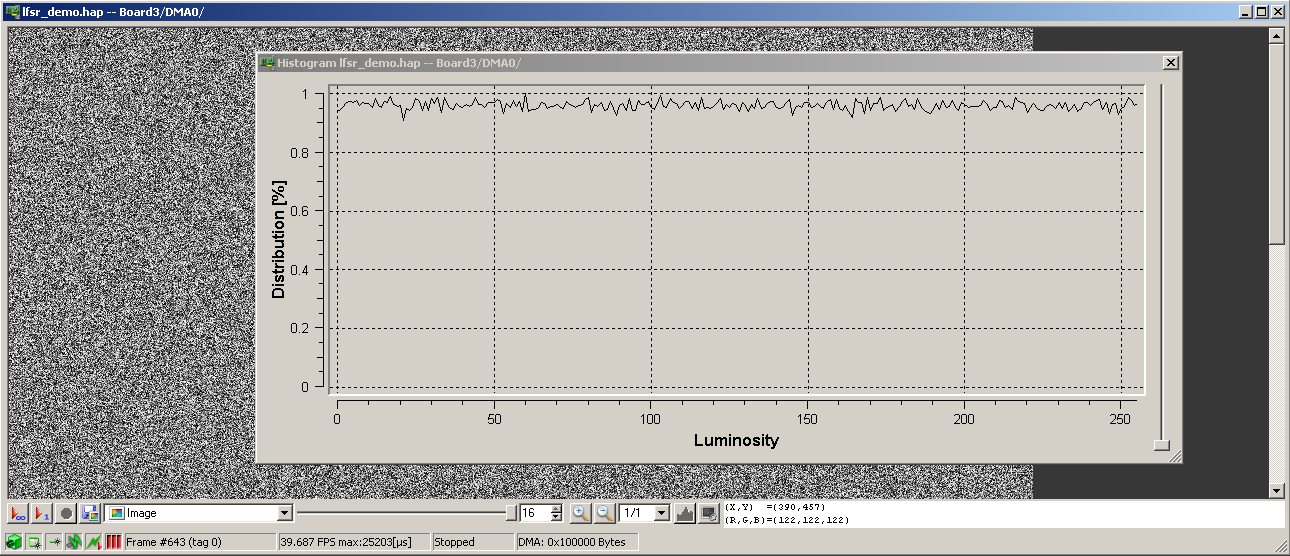2. Spectral Test:

A spectral test tests the generator on serial correlation. No patterns should be visible in the generated sequences. Bad generators show hyperplanes in the two- or three-dimensional space. The VisualApplets generator does not show any hyperplanes: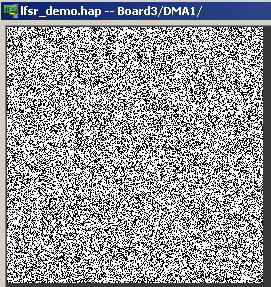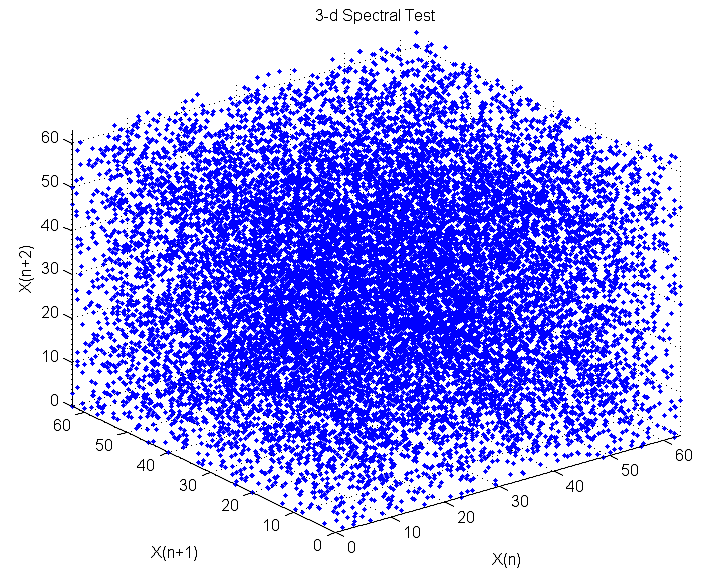An example of a bad generator is shown in the next figure. It is the famous RANDU Generator which is mostly used for the rand() function in C programming languages.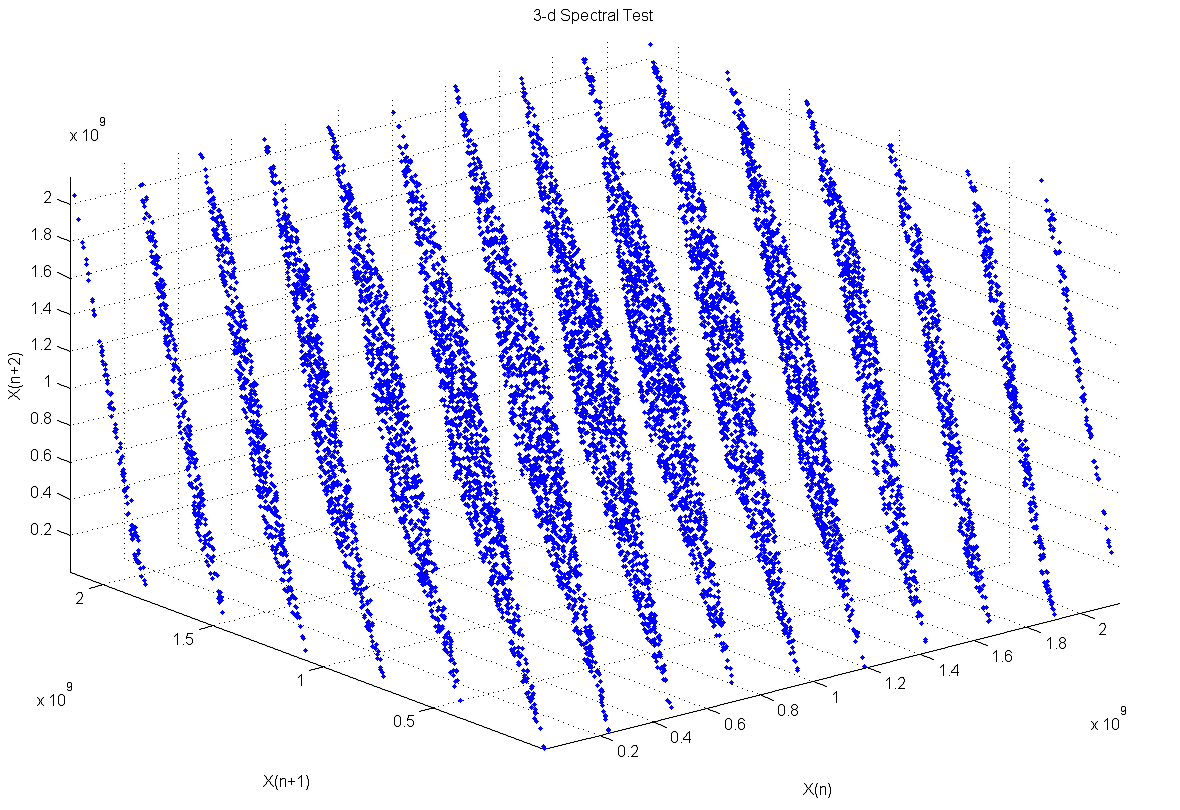### VisualApplets Simulations with PseudoRandomNumberGen

The operator can fully be simulated in VisualApplets. However, the non-deterministic hardware implementation cannot be implemented in software for simulation. Therefore, a very simple random number generator with bad quality is used in the simulation. (rand() function of the MS VisualStudio) If seeds are not changed, the operator will always generate the same sequences.

### I/O Properties

Property Value
Operator Type O

Bit Width any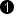[1, 64]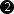Arithmetic {unsigned, signed} unsigned
Parallelism any as I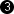Kernel Columns any 1
Kernel Rows any 1
Img Protocol {VALT_IMAGE2D, VALT_LINE1D, VALT_PIXEL0D, VALT_SIGNAL} as I
Color Format any any
Color Flavor any any
Max. Img Width any as I
Max. Img Height any as I
 The range of the input bit width is [1, 64] for unsigned gray values. For signed inputs, the range is [2, 64]. For unsigned color inputs, the range is [3, 63] and for signed color, the range is [6, 63].

### Parameters

Min_LFSR_Length
Type static parameter
Default random
Range [3, 168]

This parameter specifies the length of the shortest LFSR. Higher values cause a higher resource consumption.

Note the follwing constraint:

Seed0
Default random
Range [0, 2^64 - 1]

As explained in the operator introduction, the seeds are used to pre-initialize the LFSRs. On operator instantiation these values are initialized using a software generated random number. The values can be chnaged during acquisition. The values of all three parameters are latched when parameter Seed2 is modified.

Seed1